Next: POSITIVE REAL FUNCTIONS Up: One-sided functions Previous: MINIMUM PHASE

# FILTERS IN PARALLEL

We have seen that in a cascade of filters the filter polynomials are multiplied together. One might conceive of adding two polynomials A(Z) and G(Z) when they correspond to filters which operate in parallel. See Figure 10.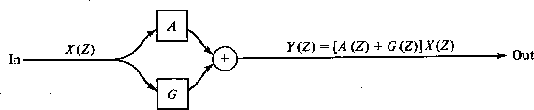2-10
Figure 10
Filters operating in parallel.

When filters operate in parallel their Z transforms add together. We have seen that a cascade of filters is minimum phase if, and only if, each element of the product is minimum phase. Now we will see a sufficient (but not necessary) condition that the sum A(Z) + G(Z) be minimum phase. First of all, let us assume that A(Z) is minimum phase. Then we may write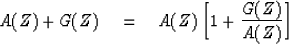(13)
The question whether A(Z) + G(Z) is minimum phase is now reduced to determining whether A(Z) and 1 + G(Z)/A(Z) are both minimum phase. We have assumed that A(Z) is minimum phase. Before we ask whether 1 + G(Z)/A(Z) is minimum phase we need to be sure that it is causal. Since 1/A(Z) is expandable in positive powers of Z only, then G(Z)/A(Z) is also causal. We will next see that a sufficient condition for 1 + G(Z)/A(Z) to be minimum phase is that the spectrum of A exceeds that of G at all frequencies. In other words, for any real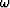,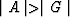. Thus, if we plot the curve of G(Z)/A(Z) in the complex plane, for real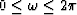it lies everywhere inside the unit circle. Now if we add unity --getting 1 + G(Z)/A(Z), the curve will always have a positive real part. See Figure 11.

 2-11 Figure 11 Phase of a positive real function lies between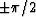.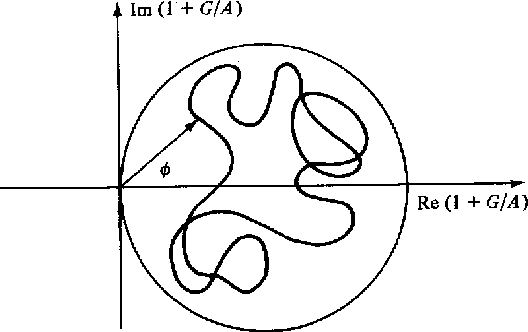Since the curve cannot enclose the origin, the phase must be that of a minimum-phase function. In words, You can add garbage to a minimum-phase wavelet if you do not add too much." This somewhat abstract theorem has an immediate physical consequence. Suppose a wave characterized by a minimum phase A(Z) is emitted from a source and detected at a receiver some time later. At a still later time an echo bounces off a nearby object and is also detected at the receiver. The receiver sees the signal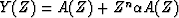where n measures the delay from the first arrival to the echo and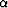represents the amplitude attenuation of the echo. To see that Y(Z) is minimum phase, we note that the magnitude of Zn is unity and that the reflection coefficientmust be less than unity (to avoid perpetual motion) so that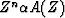takes the role of G(Z). Thus a minimum-phase wave along with its echo is minimum phase. We will later consider wave propagation situations with echoes of the echoes ad infinitum.

## EXERCISES:

1. Find two nonminimum-phase wavelets whose sum is minimum phase.
2. Let A(Z) be a minimum-phase polynomial of degree N. Let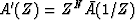. Locate in the complex Z plane the roots of A'(Z). A'(Z) is called maximum phase. [HINT: Work the simple case A(Z) = a0 + a1Z first.]
3. Suppose A(Z) is maximum phase and that the degree of G(Z) is less than or equal to the degree of A(Z). Assume.Show that A(Z) + G(Z) is maximum phase.
4. Let A(Z) be minimum phase. Where are the roots of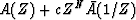in the three cases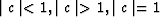? (HINT: The roots of a polynomial are continuous functions of the polynomial coefficients.)Next: POSITIVE REAL FUNCTIONS Up: One-sided functions Previous: MINIMUM PHASE
Stanford Exploration Project
10/30/1997